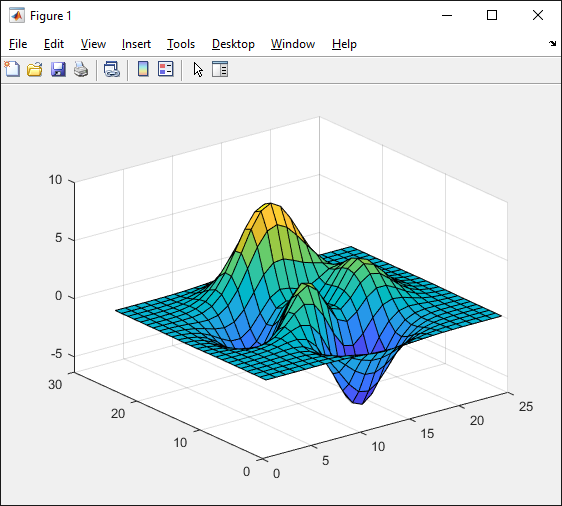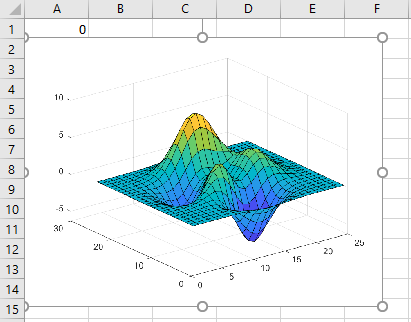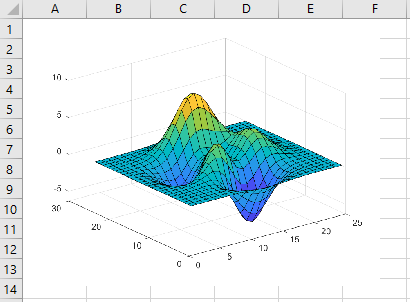MLGetFigure

Import current MATLAB figure into Microsoft Excel worksheet

Description

example

= MLGetFigure(width, height) imports the current MATLAB® figure into an Excel® worksheet, placing the top-left corner of the figure in the current worksheet cell. Specify the normalized width and height of the figure in Excel. Use this syntax when working directly in a worksheet.

example

MLGetFigure width, height imports the current MATLAB figure into an Excel worksheet, placing the top-left corner of the figure in the current worksheet cell. Use this syntax in a VBA macro.

out = MLGetFigure(width,height) lets you find errors when executing MLGetFigure in a VBA macro. If MLGetFigure fails, then out is a string containing an error code. Otherwise, out is 0.

Examples

collapse all

After you create a figure in MATLAB, import the figure into an Excel worksheet.

Note

If you use Excel 2007 or 2010, the width and height of the imported figure will be a quarter of the size of the original figure.

Create a wireframe mesh in MATLAB using the peaks and surf functions. The figure window displays a wireframe mesh.

z = peaks(25);
surf(z)Open Excel and make sure cell A1 is selected in the worksheet. Import the current figure into the worksheet using the MLGetFigure function. Enter this text in the cell and press Enter.

= MLGetFigure(.8, .8)

The MLGetFigure function imports the current figure into the worksheet, placing the top-left corner of the figure in the selected cell.After you create a figure in MATLAB, import the figure into an Excel worksheet using a VBA macro.

Create a wireframe mesh in MATLAB using the peaks and surf functions. The figure window displays a wireframe mesh.

z = peaks(25);
surf(z)On the Developer tab in Excel, click in the Code group. The Visual Basic® Editor window opens.

Select Insert > Module to insert a new module. In the Module1 window, enter this VBA code containing a macro named MyFigure.

Sub MyFigure()
MLGetFigure 0.8, 0.8
MatlabRequest
End Sub

The MyFigure macro uses the MLGetFigure function to import the current figure into the worksheet. MatlabRequest initializes internal Spreadsheet Link™ variables and enables MLGetFigure to function in a subroutine.

For details about working with modules, see Excel Help.

Select cell A1 for the position of the figure. Run the macro by clicking button on the VBA toolbar. For details about running macros, see Excel Help.

The MLGetFigure function imports the current figure into the worksheet, placing the top-left corner of the figure in the selected cell.Input Arguments

collapse all

Width (in normalized units) of the MATLAB figure when imported into an Excel worksheet, specified as a real number.

Example: 0.5

Height (in normalized units) of the MATLAB figure when imported into an Excel worksheet, specified as a real number.

Example: 0.5

Tips

• If you use Microsoft® Excel 2007 or 2010, MLGetFigure scales the imported figure by the product of width and height along both dimensions.

• If worksheet calculation mode is automatic, the software executes MLGetFigure when you enter the formula in a cell. If worksheet calculation mode is manual, enter the MLGetFigure function in a cell, then press F9 to execute it. Note that pressing F9 can also execute other worksheet functions and generate unpredictable results.

• If you use MLGetFigure in a macro subroutine, enter MatlabRequest on the line after MLGetFigure. The execution of MatlabRequest initializes internal Spreadsheet Link variables and enables MLGetFigure to function in a subroutine. Do not include MatlabRequest in a macro function unless the function is called from a subroutine.

• To work with VBA code in Excel with Spreadsheet Link, you must enable Spreadsheet Link as a reference in the Microsoft Visual Basic Editor. For details, see Installation.